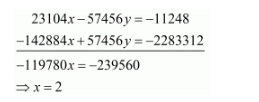# Solve the following systems of equations:

Question:

Solve the following systems of equations:

$152 x-378 y=-74$

$-378 x+152 y=-604$

Solution:

The given equations are:

$152 x-378 y=-74 \ldots(i)$

$-378 x+152 y=-604 \ldots(i i)$

Multiply equation $(i)$ by 152 and equation $(i i)$ by 378 and add both equations we getPut the value of $x$ in equation $(i)$, we get

$152 \times 2-378 y=-74$

$\Rightarrow-378 y=-378$

$\Rightarrow y=1$

Hence the value of $x=2$ and $y=1$